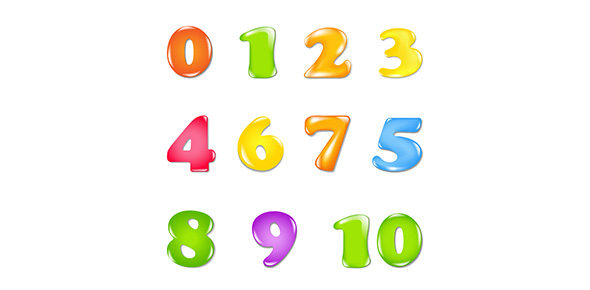# Number, Symbols, And Spacing Quiz

16 Questions | Total Attempts: 27SettingsA good knowledge of use of number, symbols, and spacing help one to write meaningful sentences to convey the right information to the recipient. The test below is designed to test your knowledge in numbers and use of spacing and symbols. Take it up and gauge your knowledge. All the best!

Related Topics
• 1.
My teacher name is mrs. Well. What should I do to correct her name?
• A.

Press the space bar

• B.

Hold the shift key

• C.

Hit the tab key

• D.

Hit the cap lock

• 2.
Susan was type a document and she wanted to leave one blank line. How many times ?
• A.

One time

• B.

Two times

• C.

3 times

• D.

4 times

• 3.
Double space contains.
• A.

One blank line

• B.

No blank line

• C.

3 blank lines

• D.

2 blank lines

• 4.
A standard word contains 5 stokes; keying 60 stokes in one minute equals a GWAM (gross words a minute) score of
• A.

25

• B.

10

• C.

12

• D.

15

• 5.
• A.

A space

• B.

Possession

• C.

A quotation

• D.

Pounds

• 6.
• A.

One time

• B.

Two time

• C.

Zero times

• D.

Three times

• 7.
To key a dash (--), combine
• A.

Two spaces

• B.

Two hyphens

• C.

Two slashes

• D.

Two apostrophes

• 8.
The ( /) symbol is call a(n)
• A.

Ampersand

• B.

Slash

• C.

Diagonal

• D.

Asterisk

• 9.
After a period following an initial or an abbreviation, space
• A.

One time

• B.

Zero time

• C.

Two time

• D.

Three time

• 10.
To key a series of uppercase (capital) letters, use the
• A.

Shift key

• B.

Caps lock key

• C.

Space bar

• D.

None

• 11.
The percent sign (%) is followed
• A.

One space

• B.

No space

• C.

Two spaces

• D.

Three spaces

• 12.
The symbol & is called a(n)
• A.

Diagonal

• B.

Asterisk

• C.

Ampersand

• D.

Slash

• 13.
The symbol * is called a(n)
• A.

Diagonal

• B.

Asterisk

• C.

Ampersand

• D.

Slash

• 14.
The hyphen (-) is followed
• A.

With no space

• B.

By one space

• C.

By two spaces

• D.

By three spaces

• 15.
The beginning quotation mark (“) is followed
• A.

With no space

• B.

By one space

• C.

By two spaces

• D.

By three spaces

• 16.
The right parenthesis is followed
• A.

With no space

• B.

By one space

• C.

By two spaces

• D.

By three spaces